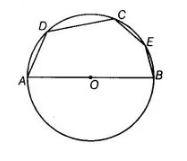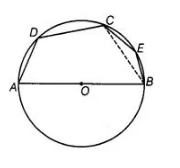# In figure, if AOB is a diameter`
Question:

In figure, if AOB is a diameter and ∠ADC = 120°, then ∠CAB = 30°.Solution:

True
Join CA and CB.[sum of opposite angles of cyclic quadrilateral is 180°]

=>∠CBA = 180° -120° = 60° [∴ ∠ADC = 120°]

In ΔACB, ∠CAB + ∠CBA + ∠ACB = 180°

[by angle sum property of a triangle]

∠CAB + 60°+ 90°= 180°

[triangle formed from diameter to the circle is 90° i.e., ∠ACB = 90°)

=> ∠CAB = 180° – 150° = 30°.